9 Financial Mathematics

# 9.5 Loans and Mortgages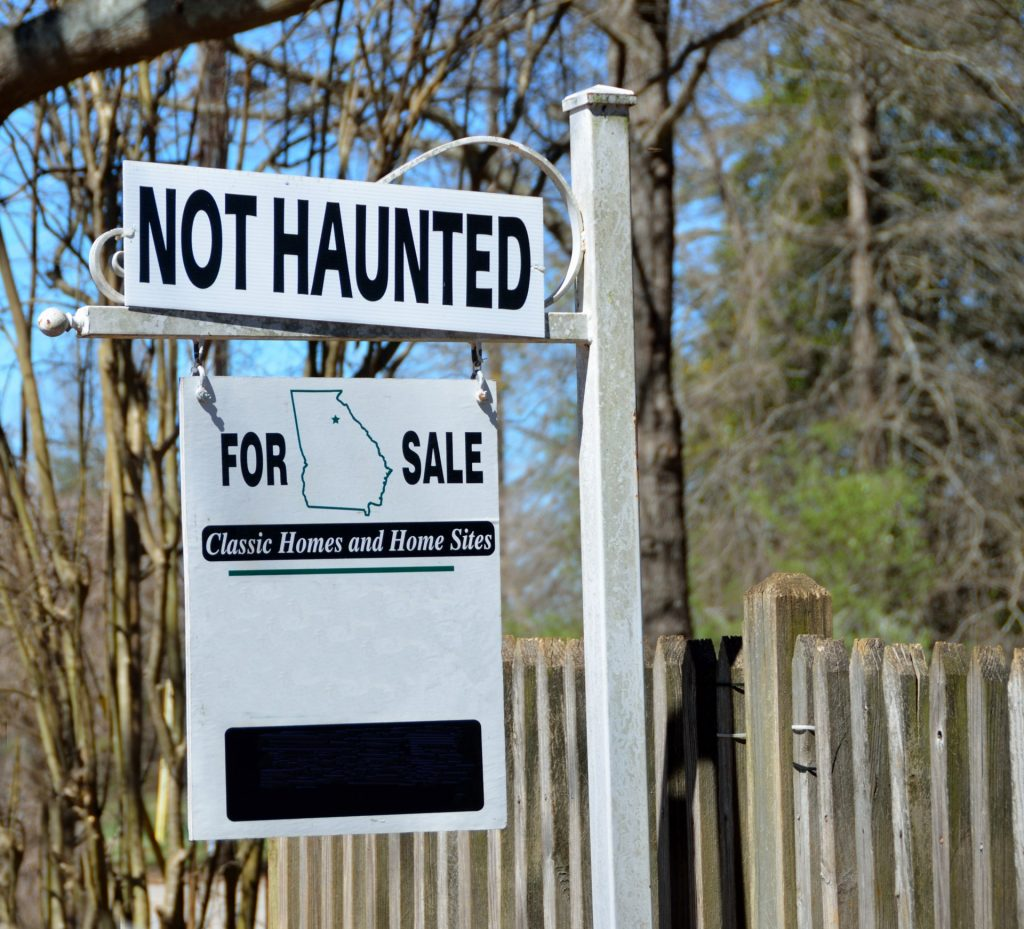Learning Objectives

By the end of this section it is expected that you will be able to:

• Determine the periodic payments on an installment loan
• Determine the amount financed and the finance charge on an installment loan
• Determine the payments and finance charge on a mortgage

# Installment Loans

A loan is something that is borrowed. In the case where this is a sum of money the amount that will be paid by the borrower will  include the original amount plus interest.

Some loans require full payment  on the maturity date of the loan.  The maturity date is when all principal and/or interest must be repaid to the the lender. Consider a one year loan of $1000 at a simple interest rate of 5%. At the end of one year (the maturity date) the borrower will pay back the original$1000 plus the interest of $50 for a total of$1050.

For major purchases such as vehicles or furniture there is a different type of loan, called the installment loan. The average consumer cannot afford to pay $25000 or more for a new vehicle and they may not want to wait three or four years until they have saved enough money to do so. The qualifying consumer has the option of paying for the item with an installment loan. Installment loans do not require full repayment of the loan on a specific date. With an installment loan the borrower is required to make regular (installment) payments until the loan is paid off. Each installment payment will include an interest charge. An installment loan can vary in length from a few years to perhaps twenty years or more (in the case of real estate). Consider an installment loan for a$4000 television. The purchaser takes out a $4000 loan with a four-year term at an interest rate of 4.5%. The monthly installment payments will be$91.21. Although the television has a purchase price of $4000, the total cost to the purchaser will be more than$4000. The total of the installment payments will be:

Total Installment Payments = Number of Installment Payments x Payment Amount =

4 years x 12 payments/year x $91.21/mth =$4378.08

The $4000 television ends up costing$4378.08 because the consumer is charged interest. Each payment includes an interest component that adds to the overall cost of the item. The total of the interest charges is referred to as the finance charge on the loan.

Finance Charge

The finance charge is the sum of the interest charges on a loan. These interest charges are embedded in the installment payments. To calculate the finance charge:

Finance Charge = Total Installment Payments – Loan Amount

= (Number of Installment Payments x Payment Amount) – Loan Amount

For the $4000 television the finance charge will be calculated as follows: Finance charge = Total Installment Payments – Loan Amount = (4 years x 12 payments/year x$91.21/payment) – $4000 =$4378.08 – $4000 =$378.08

Over the 4-year term of the loan the purchaser will have paid the $4000 loan amount plus an additional$378.08 in interest (the finance charge).

Sometimes the borrower will make an initial payment at the time of purchase. This is called a down payment. When a down payment is made the remaining amount is the amount financed or the  loan amount.

Amount Financed

The amount financed or loan amount is the purchase price of the item less any down payment:

Amount Financed = Purchase Price – Down Payment

Consider the $4000 television. Assume the purchaser makes a down payment of$1500.

The amount financed is:  Purchase Price – Down Payment = $4000 –$1500 = $2500. In this case the purchaser borrows$2500 rather than $4000. The amount financed is therefore$2500. Assuming the same 4-year term and an interest rate of 4.5%, the installment payments on the $2500 will be reduced to$57.01 per month. In this case the finance charge will be calculated as follows:

Finance charge = Total Installment Payments – Loan Amount =

(4 years x 12 payments/year x $57.01/payment) –$2500 = $2736.48 –$2500 = $236.48 With the down payment of$2500 the total finance charges will be reduced to $236.48 from$378.08.

The total cost of the television to the purchaser will be:

Purchase Price + Finance Charge

= $4000 +$236.48 = $4236.48 Alternatively we can calculate: Total Installment Payment + Down Payment =$2736.48 + $1500 =$4236.48

As one can see, the finance charges are a hidden but added cost. This cost will become more pronounced with more expensive purchases such as with real estate.

Installment Loan Terminology

Total Installment Payments = Number of payments x Payment Amount

Finance Charge = Total Installment Payments – Loan Amount

Amount Financed or Loan Amount = Purchase Price of Item  – Down payment

EXAMPLE 1

Paul purchased a home entertainment system at a total cost of $6000. He obtained a 3 year loan at an interest rate of 7.5%. His monthly payments will be$186.64 over three years.

a) State the amount financed.

b) Determine the total installment payments.

c) Determine the finance charge.

Solution

a)  Since there was no down payment the amount financed (or loan amount) will be $6000. b) The total installment payments will be: Number of payments x Payment Amount = 3 years x 12 payments/year x$186.64

= $6719.04 c) Finance Charge = Total installment payments – Loan Amount =$6719.04 – $6000 =$719.04

TRY IT 1

Cassie purchased a new washer and dryer at a total cost of $3800. She obtained a 4 year loan at an interest rate of 6.2%. Her monthly payments will be$89.59 over four years.

a) State the amount financed.

b) Determine the the total installment payments.

c) Determine the finance charge.

a)  $3800.00 b)$4300.32    c) $500.32 EXAMPLE 2 Mike purchased a home entertainment system at a total cost of$6000. He made a down payment of $1800 and to pay the balance he obtained a 3 year loan at an interest rate of 7.5%. His monthly payments will be$130.65 over three years.

a) State the amount financed.

b) Determine the total installment payments.

c) Determine the finance charge.

d)  Determine the total amount that Mike paid for the home entertainment system

Solution

a)  Amount Financed = Cost of Item – Down Payment

=  $6000 –$1800 = $4200 b) The total installment payments will be: Number of payments x Payment Amount = 3 years x 12 payments/year x$130.65

= $4703.40 c) Finance Charge = Total installment payments – Loan Amount =$4703.40 – $4200 =$503.40

d) Total paid = Purchase Price + Finance Charge = $6000 +$503.40 = $6503.40 TRY IT 2 Carl purchased a new washer and dryer at a total cost of$3800. He made a down payment of $1500 and obtained a 2 year loan for the remaining amount at an interest rate of 6.2%. His monthly payments will be$102.14 over two years.

a) State the amount financed.

b) Determine the total installment payments.

c) Determine the finance charge.

d)   Determine the total amount that Carl paid for the washer and dryer.

a) $2300.00 b)$2451.36

c)  $151.36 d)$3951.36

# Loan Payments

When consumers obtain installment loans they often just trust the lender to determine the installment (periodic)  loan payments. In Example1 Paul purchased a home entertainment system at a total cost of $6000. He obtained a three year loan at an interest rate of 7.5%. If Paul attempts to calculate his monthly payment by simply dividing the loan amount by the number of payments he will underestimate his monthly payment as he has ignored the interest component:$6000 ÷ 36 = $166.67 Paul’s actual monthly payment of$186.64 is slightly higher than Paul’s estimate because of  the interest component.
The actual amount of a periodic loan payment can be determined using a formula, a table or technology. In this section we will illustrate the use of a formula.

Periodic Payment on a Loan

The periodic payment on a loan formula is: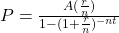P = periodic payment amount A = amount of loan r = annual interest rate (in decimal form) n = number of payments made in one year t = time (in years)

EXAMPLE 3

Refer back to the purchase of a television for $4000. The purchaser agrees to a 4 year term at an interest rate of 4.5%. a) Use the formula to determine the monthly installment payment b) Determine the total installment payments Solution a)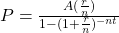where P = payment (unknown), A =$4000, r = 4.5%, n = 12, t = 4 years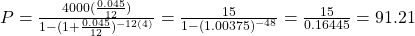The monthly payment is confirmed to be $91.21. b) Total installment payments = monthly payment amount x no. of payments$91.21 x 48= $4378.08 TRY IT 3 A dining room table set is purchased for$5600. The purchase is financed  with a 3 year loan at an interest rate of 12.5%. a) Use the formula to determine the monthly installment payment  b) Determine the total installment  payments.

Monthly payment is $187.34; Total Installment payments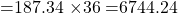EXAMPLE 4 Paul purchased a home entertainment system at a total cost of$6000. He obtained a 3 year loan at an interest rate of  7.5%.  Use the formula to determine his monthly payments. Confirm that this matches the amount in Example 1.

SolutionP = payment (unknown) where A = $6000 r = 7.5% n = 12 t = 3 years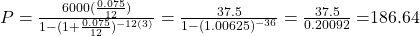The monthly payment is confirmed to be$186.64

TRY IT 4

Cassie purchased a new washer and dryer at a total cost of $3800. She obtained a 4 year loan at an interest rate of 6.2%. Use the formula to determine her monthly payments. Confirm that this matches the amount in Try It 1. Show answer Monthly payment of$89.59 is confirmed

EXAMPLE 5

Determine   a) the annual payments   b)the total installment payments  and  c) the finance charge on a 5 year loan of $5000 where payments are made annually and the interest rate is 6%. Solution a)P = payment A =$5000    r = 6%

n = 1            t = 5 years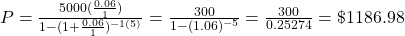The annual payment will be $1186.98. b) Total installment payments =$1186.98 x 5 = $5934.90 c) Finance charge =$5934.90 – $5000 =$934.90

TRY IT 5

Determine a) the annual payments   b) the  total installment payments  and  c) the finance charge  on a 5 year loan of $5000 where payments are made monthly and the interest rate is 6%. Show answer a) Annual payment is$96.67

b) Total Installment payments = $5800.20 c) Finance charge$800.20

Recall that interest is calculated only on the loan amount and not on any downpayment. When determining the periodic payment on an installment loan be sure to exclude the downpayment when  calculating the periodic payment.

EXAMPLE 6

Mike purchased a home entertainment system at a total cost of $6000. He made a down payment of$1800 and to pay the balance he obtained a 3 year loan at an interest rate of  7.5%.   Use the formula to determine his monthly payments. Confirm that this matches the amount provided in Example 2.

SolutionP = payment (unknown) where A = $4200 r = 7.5% n = 12 t = 3 years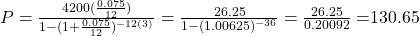The monthly payment is confirmed to be$130.65

TRY IT 6

Carl purchased a new washer and dryer at a total cost of $3800. He made a down payment of$1500 and obtained a 2 year loan for the remaining amount at an interest rate of  6.2%.  Use the formula to determine his monthly payments. Confirm that this matches the amount provided in Try It 2.

Monthly payment of $102.14 is confirmed EXAMPLE 7 Pat has decided to purchase a used vehicle that costs$12,500. He considers two options. For each option, determine a) the monthly payment  b) total installment payments  c) the finance charge for each option. What is the difference in the finance charge with the down payment?

Option 1) Paying the full amount with a 4 year loan, monthly payments, and an interest rate of 6.8%.

Option 2) He will cancel a planned trip and and instead make a  $3500 down payment on the purchase. He will pay the remaining amount with a 4 year loan, monthly payments, and an interest rate of 6.8%. Solution Option 1) a) P = unknown A =$12,500

r = 0.068        n = 12          t = 4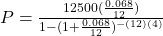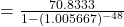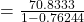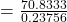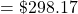payment

b) Total Installment payments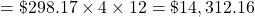c) Finance charge = Total Installment Payments – Loan Amount = $14312.16 –$12,500 = $1812.16 Option 2) a) P = unknown A =$12,500 – $3500 =$9000

r = 0.068        n = 12          t = 4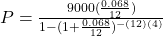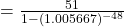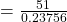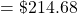payment

b) Total Installment payments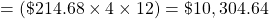c) Finance charge = Total Installment Payments – Loan Amount = $10,304.64 –$9000 = $1304.64 With a down payment there will be a savings of$507.52 on the finance charges.

TRY IT 7

Mick has decided to purchase a home entertainment system at a cost of  $9200. He considers two options. For each option determine a) the monthly payment b) total installment payments c) the finance charge for each option. What is the difference in the finance charge with the down payment? 1) Paying the full amount with a 3 year loan that offers an interest rate of 8.4%. 2) Forgoing the purchase of a new electric bike and instead makinga$2000 down payment on the bike purchase. He will pay the remaining amount with a 3 year loan at an interest rate of 8.4%.

With no down payment: a) $290 b)$10440   c) $1239.83 With a down payment a)$226.95  b)   $10170.20 c)$970.30;  With the down payment the finance charge is $269.53 less # Amortization Amortization is the process of spreading out a loan into a series of fixed payments. A portion of each payment will be applied to the interest charge and a portion will be applied to the principal amount of the loan. Although each payment is equal, the amount that applies to the interest versus the prinipal will change with each payment period. We can get a better sense of the impact that a loan payment has by examining the amortization schedule for a loan. Consider the amortization table for the installment loan in Example 5. Recall that the loan amount is$5000 at 6% for 5 years and annual payments are $1186.98. Note then that for each year the sum of the interest and principal is equivalent to the payment of$1186.98. Refer to Figure 1 for the amortization schedule of this loan.
To calculate the interest (I) we use the simple interest formula I = Prt . The principal (P) will be the beginning balance for each year. The time in years is the portion of the year for which interest is being calculated. In this example the time (t) is one year and the interest rate is 6%.
In Year 1 the interest on the loan of $5000 will be: I = Prt =$5000 x 0.06 x 1yr = $300. The periodic payment amount is$1186.98 and the portion that will go towards interest is $300. The portion that will go towards paying down the principal will be: periodic payment amount – interest =$1186.98 – $300 =$886.98.
Although the payment was $1186.98, only$886.98 will be applied to the outstanding loan amount. At the end of year 1 the remaining balance on the loan will be:
beginnining balance – portion applied to the principal
=  $5000 –$886.98 = $4113.02 In Year 2 the beginning balance on the loan is$4113.02. The interest on the loan will be:
I = Prt = $4113.02 x 0.06 x 1yr =$246.78.
Note that interest is calculated on the remaining balance of the loan, not on the original $5000. For the periodic payment of$1186.98, the portion that will go towards interest is $246.78. The portion that will go towards paying down the principal will be: periodic payment amount – interest =$1186.98 – $246.78 =$940.20.
At the end of year 2 the remaining balance on the loan will be:
beginnining balance – portion applied to the principal
=  $4113.02 –$940.20 = $3172.82 In Year 3 the beginning balance on the loan is$3172.82. The interest on the loan will be:
I = Prt = $3172.82 x 0.06 x 1yr =$190.37
The portion that will go towards paying down the principal will be:
periodic payment amount – interest
= $1186.98 –$190.37  = $996.61 At the end of year 3 the remaining balance on the loan will be: beginnining balance – portion applied to the principal =$3172.82 – $996.61 =$2176.21

The cycle repeats for five years until the loan is paid off. If we add the interest charges in the table they will total to $934.91. This is the same as the finance charge (ignoring the 1¢ difference due to rounding) that was calculated in Example 5. The amortization table illustrates that in the early periods of the loan a larger portion of the payment goes towards interest and a smaller portion contributes to paying down the principal (loan) amount. Over time a larger portion of the payment will be applied towards paying down the balance on the loan. For large purchases it can take several payment periods before the payment contributes substantailly to the principal balance of the loan. A down payment is beneficial as it will reduce the total finance charge. # Mortgages A long term loan that is used for the purchase of a house is called a mortgage. It is called a mortgage because the lending agency requires that the house be used as collateral for the loan. This means that if the mortgage holder is unable to make the payments the lender can take possession of the house. Mortgages generally tend to be for longer time periods than an installment loan and the terms of the mortgage will often change over the course of the mortgage. Take for example the purchase of a house with a twenty year mortgage. The purchaser might sign a mortgage agreement for a five year term. The mortgage agreement will include the interest rate, the frequency of payments and additional rules which may allow the mortgage holder to make lump sum payments or change the payment amount. At the end of the five year term a new agreement will be required and the conditions of the mortgage usually change. Although it is possible to do the calculations manually, that is beyond the scope of this book. We will use technology to calculate the periodic payments and interest charges and to generate an amortization schedule. Example 8 will illustrate that amortizing a mortgage is similar to amortizing other loans except that the mortgage amortization generally involves many more payment periods. EXAMPLE 8 A$400,000 home is purchased with a 20% down payment on a 20-year mortgage at a fixed interest rate of 3.4%.

a) Determine the down payment.

b) Use an online mortgage calculator to determine the monthly payment and the total interest paid.

c) Generate an annual amortization schedule.

d) Determine the total payments for one  year

e) Use the table to determine how much of the first year’s  payments will go towards interest and how much will go towards the principal.

f) Use the table to determine how much of the final year’s  payments will go towards interest and how much will go towards  the principal.

Solution:

a) The down payment will be 20% x $400,000 =$80,000.

b) The monthly payment will be $1839.47 and the total interest will be$121, 472.75.

c)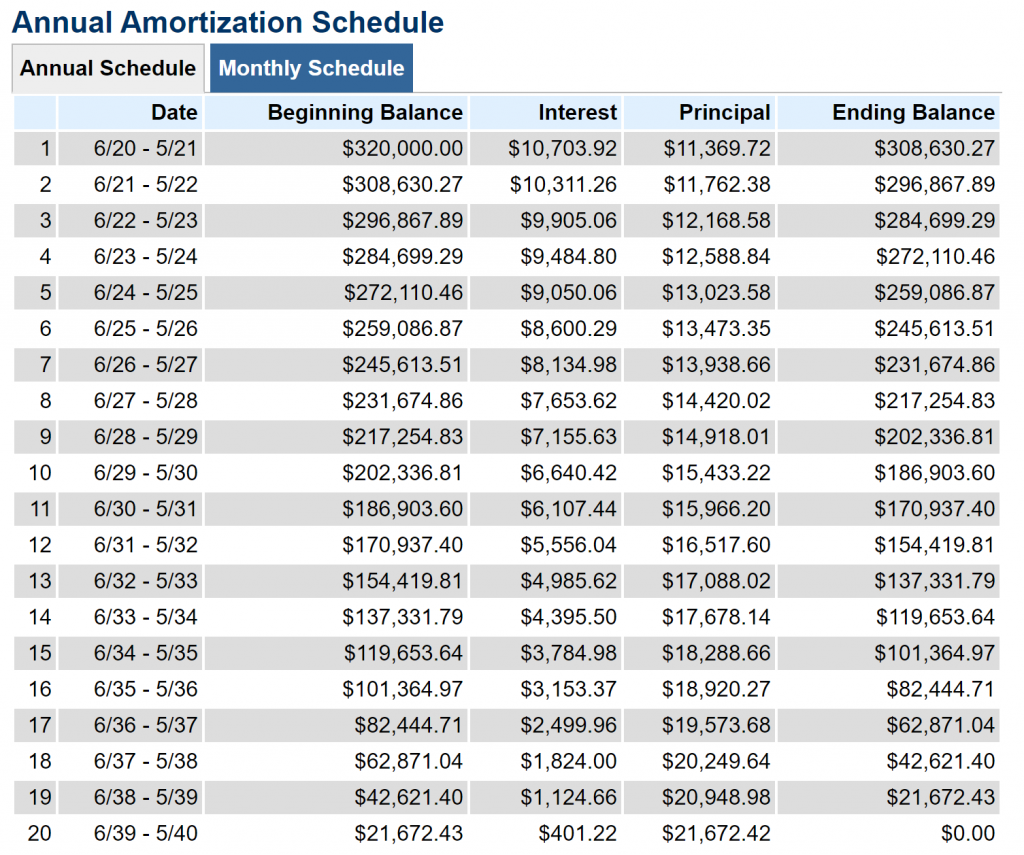d) In one year the total payments will be 12 x $1839.47 =$22,073.64.

e) Of the first year’s payments, almost half, $10,703.92, will go towards interest.$11,369.72 will go towards paying down the principal.

f) Of the final year’s payments, $401.22 will go towards interest.$21, 672.42  will go towards the principal.

TRY IT 8

A 20-year mortgage is obtained to purchase a $550,000 home with a 15% down payment at a fixed interest rate of 4.6%. a) Determine the down payment. b) Use an online mortgage calculator to determine the monthly payment and the total interest paid. c) Generate an annual amortization schedule. d) Determine the total payments for one year e) Use the table to determine how much of the first year’s payments will go towards interest and how much will go towards the principal. f) Use the table to determine how much of the final year’s payments will go towards interest and how much will go towards the principal. Show answer a) The down payment will be$82,500

b) the monthly payment will be $2982.93 and the total interest will be$248,403.36

d) In the first year the total payments will be $35,795.16. e) In the first year$21,199.84, will go to interest. $14,595.32 will go towards paying down the principal. f) In the final year$876.17 will go to interest. $34,918.99 will go towards paying down the principal. EXAMPLE 9 A young couple have received an inheritance and they now have enough money for a down payment on their first home. They plan to take out a 25 year mortgage at an interest rate of 3.8%. They are considering a new house for$750,000  or a smaller older home for $380,000. If they purchase the larger house they plan to make a 20% down payment. With the less expensive smaller house they can afford a 35% down payment. a) Use an online mortgage calculator to determine the down payment, the monthly payment and the total interest paid for each of the two houses. b) For each of the houses, what is the principal balance owing after 5 years? Solution a)$750,000 house:   $150,000 down payment;$3101.14 monthly payment;  Total interest $330,341.81$380,000 house:   $133,000 down payment;$1276.64 monthly payment;  Total interest $135,990.71 b)$750,000 house: After 5 years the balance owing is $520,767.80$380, 000 house:  After 5 years the balance owing is $214,382.74 TRY IT 9 A couple has won$50,000 in the lottery and they decide to put this towards the purchase of a vacation cottage or a house. They plan to make a 10%  down payment and are considering a 25 year mortgage at a rate of 2.9%. They are deciding between the purchase of a  cottage for  $500,000 or a house for$880,000.

a) Use an online mortgage calculator to determine the down payment, the monthly payment and the total interest paid for the cottage and for the house.

b) For each of the cottage and the house, what is the principal balance owing after 5 years?

a) Cottage: The down payment will be $50,000, the monthly payment will be$2110.62 and the total interest will be $183,185.76 House: The down payment will be$88,000, the monthly payment will be $3714.69 and the total interest will be$322,406.93

b)  Cottage: After 5 years the balance owing is $384,024.74 House: After 5 years the balance owing is$675,883.55

# Key Concepts

• For an Installment Loan:
• to determine the total installment payments:

Number of Payments x Payment Amount

• to determine the finance (interest) charge:

Total Installment Payments  – Loan Amount

• to determine the amount financed:

Purchase Price – Down Payment

• to determine the total amount paid for the item:

Purchase Price + Finance Charge

or

Total  Installment Payments + Down Payment

• to determine the periodic payment P:# Glossary

amortization

is the process of spreading out a loan into a series of fixed payments.

amount financed

is the purchase price of the item less any down payment.

finance charge

is the total of the  interest charges on a loan.

installment loan

is a type of loan that is repaid over time with a set number of scheduled payments (installments). The term of loan may be vary and could be few months or many years.

maturity date

is when all principal and/or interest must be repaid to the lender.

# 9.5 Exercise Set

1. Bette purchased a new appliance package at a total cost of $7500. She obtained a 3 year loan at an interest rate of 5.75%. Her monthly payments will be$227.32 over three years.
1. State the amount financed.
2. Determine the total installment payments.
3. Determine the finance charge.
2. Paul purchased a new vehicle at a total cost of $21,300. He obtained a 5 year loan at an interest rate of 4.2%. His monthly payments will be$394.20 over five years.
1. State the amount financed.
2. Determine the total installment payments.
3. Determine the finance charge.
3. Theresa purchased a home entertainment system at a total cost of $4300. She made a down payment of$1000 and to pay the balance she obtained a 2 year loan at an interest rate of  5.5%.  Her monthly payments will be $145.52 over two years. 1. State the amount financed. 2. Determine the total installment payments. 3. Determine the finance charge. 4. Determine the total amount that Theresa paid for the home entertainment system. 4. The Johnsons purchased a new vehicle at a total cost of$32,500. They made a down payment of $5000 and to pay the balance they obtained a 4 year loan at an interest rate of 3.6%. The monthly payments will be$616.01 over four years.
1. State the amount financed.
2. Determine the total installment payments..
3. Determine the finance charge.
4. Determine the total amount that the Johnsons paid for the vehicle.
5. Determine the monthly (periodic) payment and finance charge for each of the following installment loans.
 Annual Interest Rate Number of Years Loan Amount Monthly Payment Finance Charge 2.8% 1 $2000 4% 2$4200 5% 3 $5200 4.5% 3$8000 6.5% 4 $11,000 6. A dining room set is purchased for$2300. The purchase is financed  with a 2 year loan at an interest rate of 6.4%
1. Use the formula to determine the monthly payment
2. Determine the total installment  payments.
3. Determine the finance charge.
7. A new vehicle is purchased for $32, 000. The purchase is financed with a 5 year loan at an interest rate of 4.8%. 1. Use the formula to determine the monthly payment 2. Determine the total installment payments. 3. Determine the finance charge. 8. The Connors purchase a hot tub for a total price of$8500. They make a downpayment of $2300 and finance the remainder with a 3 year loan at an interest rate of 2.6%. 1. Determine the loan amount 2. Use the formula to determine the monthly payment 3. Determine the total installment payments. 4. Determine the finance charge. 5. How much in total did the Connors actually pay for the hot tub? 9. The Tanners purchase a small RV for a total price of$48,000. They make a downpayment of $8000 and finance the remainder with a 4 year loan at an interest rate of 3%. 1. Determine the loan amount 2. Use the formula to determine the monthly payment 3. Determine the total installment payments. 4. Determine the finance charge. 5. How much in total did the Tanners actually pay for the RV? 10. Matt borrows$4000 for 4 years at an interest rate of 5%. He will make 4 annual payments.
1. Determine the annual payment and the finance charge.
2. Complete the following amortization table for the loan.
 Year Beginning Balance Interest Payment towards the Principal = Payment – Interest End Balance 1 $4000$200 2 3 4
3. Confirm the finance charge by totalling the interest column.
11. Kate purchases an electric bike for $4800 and she makes a down payment of$2200. She takes out a one year loan at 3.2% to pay the balance owing in monthly payments.
1. Determine the amount of the loan
2. Determine the monthly payment on the loan.
3. Determine the total installment payments
4. Determine the finance charge.
5. Complete the following amortization table for the first four months of the loan. (Hint: When calculating simple interest the time (t) will be 1/12 of  a year).
 Year Beginning Balance Interest Payment towards the Principal = Payment – Interest End Balance 1 2 3 4
6. How much did Kate actually pay for the bike?
12. You purchase a kayak for $4800 and take out a 3 year loan with monthly payments at an annual interest rate of 3.5%. You are pondering whether to put$2000 down or go on a holiday with that $2000. 1. Assuming no down payment, determine the monthly payment, total installment payments, and finance charge. 2. Assuming a down payment of$2000, determine the monthly payment, total installment payments,  and finance charge.
3. What is the difference in finance charges between the two options?
13. Nick purchases a used motorbike for $12,000 and takes out a 4 year loan with monthly payments at an annual interest rate of 5%. 1. Determine the payment, total installment payments, and finance charge with no down payment. 2. Determine the payment, total installment payments, and finance charge with a down payment of$4000.
3. What is the difference in finance charges between the two options?
14. A $350,000 home is purchased with a 20 year mortgage at a fixed interest rate of 3.4% and a down payment of 10%. 1. Use an online mortgage calculator to determine the down payment, the monthly payment and the total interest paid. 2. Determine the total payments for one year. 3. Generate an amortization schedule and determine how much of the first year’s payments will go towards principle and how much will go towards interest. 4. Generate an amortization schedule and determine how much of the final year’s payments will go towards principle and how much will go towards interest. 15. A$350,000 home is purchased with a 20 year mortgage at a fixed interest rate of 3.4% and a  20% down payment.
1. Use an online mortgage calculator to determine the down payment, the monthly payment and the total interest paid
2. Compare your answers for #14 and #15 Part a). What was the impact on the monthly payment and the total interest charges when the down payment was doubled?
3. Determine the total payments for one year.
1. A $650,000 home is purchased with a 10% down payment on a 25 year mortgage at a fixed interest rate of 4.2%. Use an online mortgage calculator to determine the down payment, the monthly payment and the total interest paid. 2. A$650,000 home is purchased with a 10% down payment on a 25 year mortgage at a fixed interest rate of 2.2%.  Use an online mortgage calculator to determine the down payment, the monthly payment and the total interest paid
3. Compare your answers for parts a) and b). How does the lower interest rate impact the total interest paid?

1. $7500 2.$8183.52
3. $683.52 1.$21,300
2. $23,652 3.$2352
1. $3300 2.$3492.48
3. $192.48 4.$4492.48
1. $27,500 2.$29,568.48
3. $2068.48 4.$34,568.48
1.  Annual Interest Rate Number of Years Loan Amount Monthly Payment Finance Charge 2.8% 1 $2000$169.21 $30.52 4% 2$4200 $182.38$177.12 5% 3 $5200$155.85 $410.60 4.5% 3$8000 $237.98$567.28 6.5% 4 $11,000$260.86 $1521.28 1.$102.35
2. $2456.40 3.$156.40
1. $600.95 2.$36,057
3. $4057 1.$6200
2. $179.21 3.$6451.56
4. $251.56 5.$8751.56
1. $40,000 2.$885.37
3. $42,497.76 4.$2497.76
5. $50,497.76 1. Annual Payment =$1128.05. Finance Charge =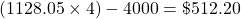2.  Year Beginning Balance Interest Payment towards the       Principal (Balance) = Payment – Interest End Balance 1 4000 200 1128.05 – 200 = 928.05 4000 – 928.05 = 3071.95 2 3071.95 153.60 1128.05 – 153.60 = 974.45 3071.95 – 974.45 = 2097.50 3 2097.50 104.87 1128.05 – 104.87 = 1023.18 2097.50 – 1023.28 = 1074.32 4 1074.32 53.72 1128.05 – 53.72 = 1074.33 1074.32 – 1074.33 = 0
3. Interest = $512.19 (rounding difference of 1¢) 1. Loan Amount =$2600
2. Monthly Payment = $220.44 3. Total Installment payments =$2645.28
4. Finance Charge =  $45.28 5.  Year Beginning Balance Interest (t = 1/12 year) Payment towards the Principal (Balance) = Payment – Interest End Balance 1 2600 6.93 220.44 – 6.93 = 213.51 2600 – 213.51 = 2386.49 2 2386.49 6.36 220.44 – 6.36 = 214.08 2386.49 – 214.08 = 2172.41 3 2172.41 5.79 220.44 – 5.79 = 214.65 2172.41 – 214.65 = 1957.76 4 1957.76 5.22 220.44 – 5.22 = 215.22 1957.76 – 215.22 = 1742.54 6.$4845.28
1. Payment = $140.65; Total Installment Payments =$5063.40;  Finance Charge = $263.40 2. Payment =$82.05;   Total Installment Payments = $2953.65 Finance Charge =$153.65
3. $109.75 less with a down payment 1. Payment =$276.35;  Total  Installment Payments = $13264.80; Finance Charge =$1264.80
2. Payment = $184.23; Total Installment Payments =$8843.04;  Finance Charge = $843.04 3.$421.76 less with a down payment
1. down payment of $35,000; monthly payment of$1810.73;  total interest paid  $119,574.74 2. total payments for one year$21,728.76
3. $10,536.66 towards interest and$11,192.10 towards principal.
4. $394.95 towards interest and$21,333.81 towards principal.
1. down payment of $70,000; monthly payment of$1609.54;  total interest paid  $106,288.66 2. With the downpayment being doubled, the monthly payment was reduced by close to$200 and the total interest paid was reduced by more than $13,000. 3.$19,314.48
1. down payment of $65,000; monthly payment of$3152.81;  total interest paid  $360,843.77 2. down payment of$65,000; monthly payment of $2536.90; total interest paid$176,070.84
3. the total interest is almost \$200,000 less!